RS Aggarwal Class 8 Solutions Chapter 2 - Exponents

RS Aggarwal Class 8 Chapter 2 - Exponents Solutions Free PDF

This chapter is designed to teach students about exponents and how to calculate a product of a number when it has a exponent over it. Learning the concepts of exponents helps students to calculate faster when complex calculations are there. Learn the concepts of exponents by solving the RS Aggarwal questions. Solving the RS Aggarwal questions will help the students to understand the concepts easily. To help students we have also provided the RS Aggarwal class 8 solutions chapter 2 exponents.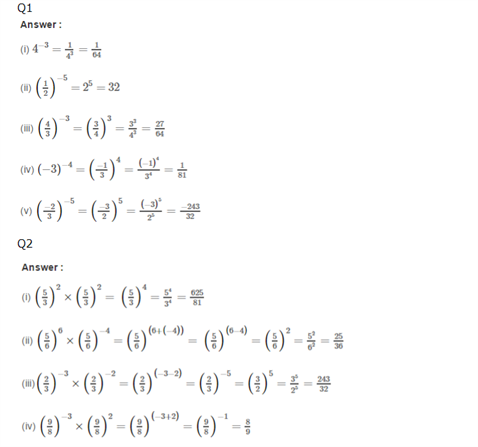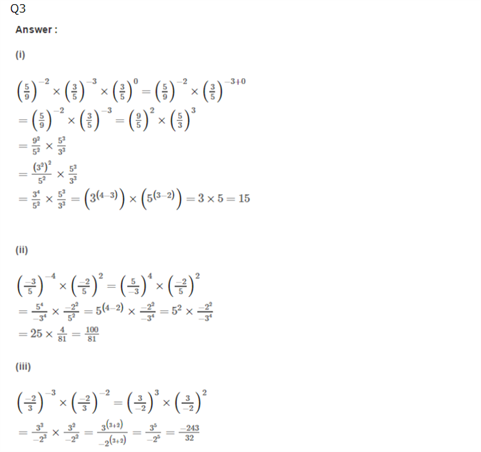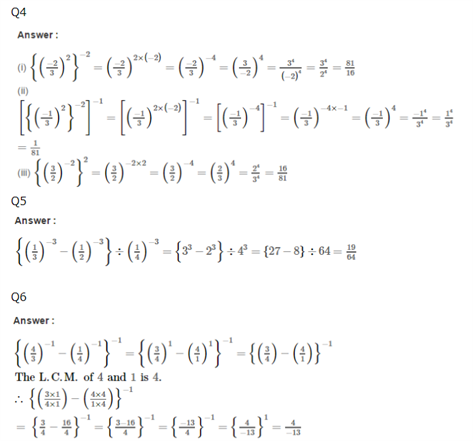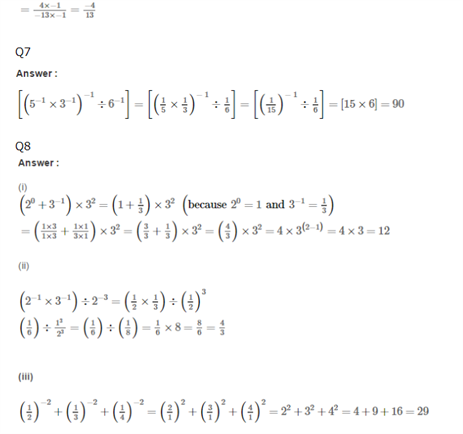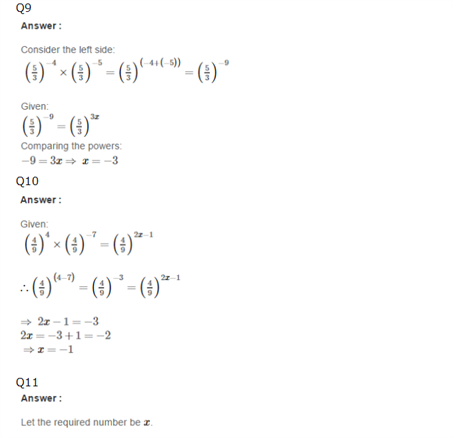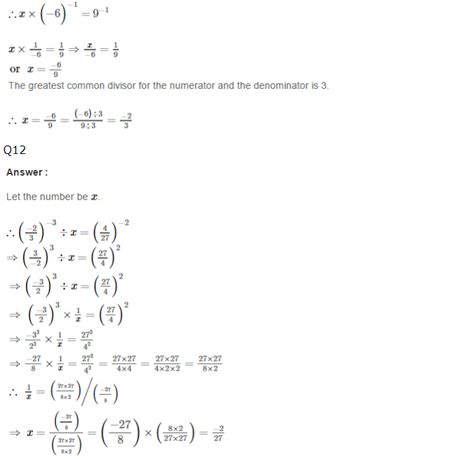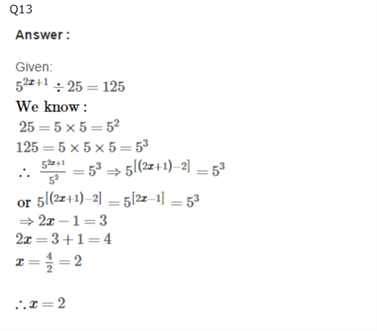Practise This Question

Between any two given rational numbers, there are countless rational numbers.